## Self GMD and Mutual GMD:

Self GMD and Mutual GMD – The use of self geometrical mean distance (abbreviated as Self GMD) and mutual geometrical mean distance (Mutual GMD) simplifies the inductance calculations, particularly relating to multiconduc­tor arrangements. The symbols used for these are respectively Ds and Dm. We shall briefly discuss these terms.

Self GMD (Ds): In order to have concept of Self GMD (also sometimes called Geometri­cal mean radius ; GMR), consider the expression for inductance per conductor per metre already derived in Art. 9.5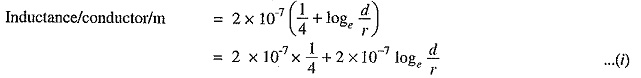In this expression, the term 2 x 10-7 x (1/4) is the inductance due to flux within the solid conduc­tor. For many purposes, it is desirable to eliminate this term by the introduction of a concept called Self GMD or GMR. If we replace the original solid conductor by an equivalent hollow cylinder with extremely thin walls, the current is confined to the conductor surface and internal conductor flux linkage would be almost zero. Consequently, inductance due to internal flux would be zero and the term 2 x 107 x (1/4) shall be eliminated. The radius of ills equivalent hollow cylinder must he sufficiently smaller than the physical radius of the conductor to allow room for enough additional flux to compensate for the absence of internal flux linkage. It can be proved mathematically that for a solid round conductor of radius r; the self-GMD or GMR = 0.7788 r. Using self-GMD, the eq. (i) becomes :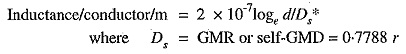It may be noted that Self GMD of a conductor depends upon the size and shape of the conductor and is independent of the spacing between the, conductors.

Mutual GMD: The Mutual GMD is the geometrical mean of the distances form one con­ductor to the other and, therefore, must be between the largest and smallest such distance. In fact, Mutual GMD simply represents the equivalent geometrical spacing.

The Mutual GMD between two conductors (assuming that spacing between conductors is large compared to the diameter of each conductor) is equal to the distance between their centres i.e.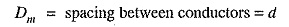For a single circuit 3-Φ line, the Mutual GMD is equal to the equivalent equilateral spacing i.e. (d1d2d3)1/3.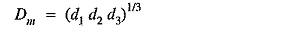The principle of geometrical mean distances can be most profitably employed to 3-Φ double circuit lines. Consider the conductor arrangement of the double circuit shown in Fig. 9.10. Suppose the sad us ,of each conductor is r.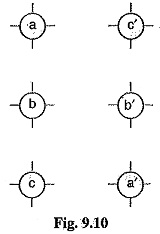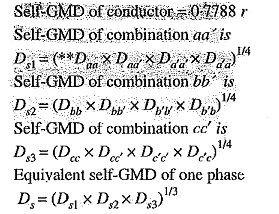The value of Ds is the same for all the phases as each conductor has the same radius.

Mutual GMD between phases A and B is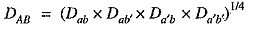Mutual GMD between phases B and C is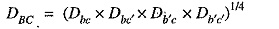Mutual GMDD between phases C and A is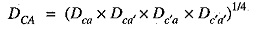Equivalent Mutual GMD,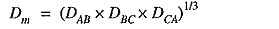It is worthwhile to note that Mutual GMD depends only upon the spacing and is substantially independent of the exact size, shape and orientation of the conductor.

### Inductance Formulas M Terms of GMD

The inductance formulas developed in the previous articles can be conveniently expressed in terms of geometrical mean distances.

1.Single phase line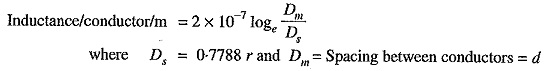2.Single circuit 3-Φ line3.Double circuit 3-Φ line

Scroll to Top